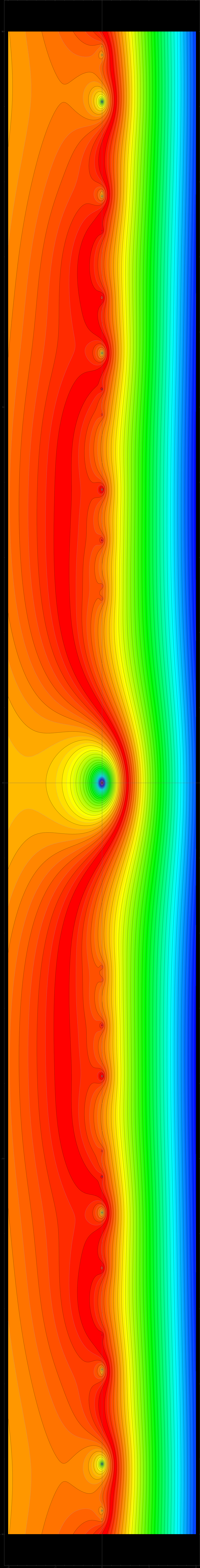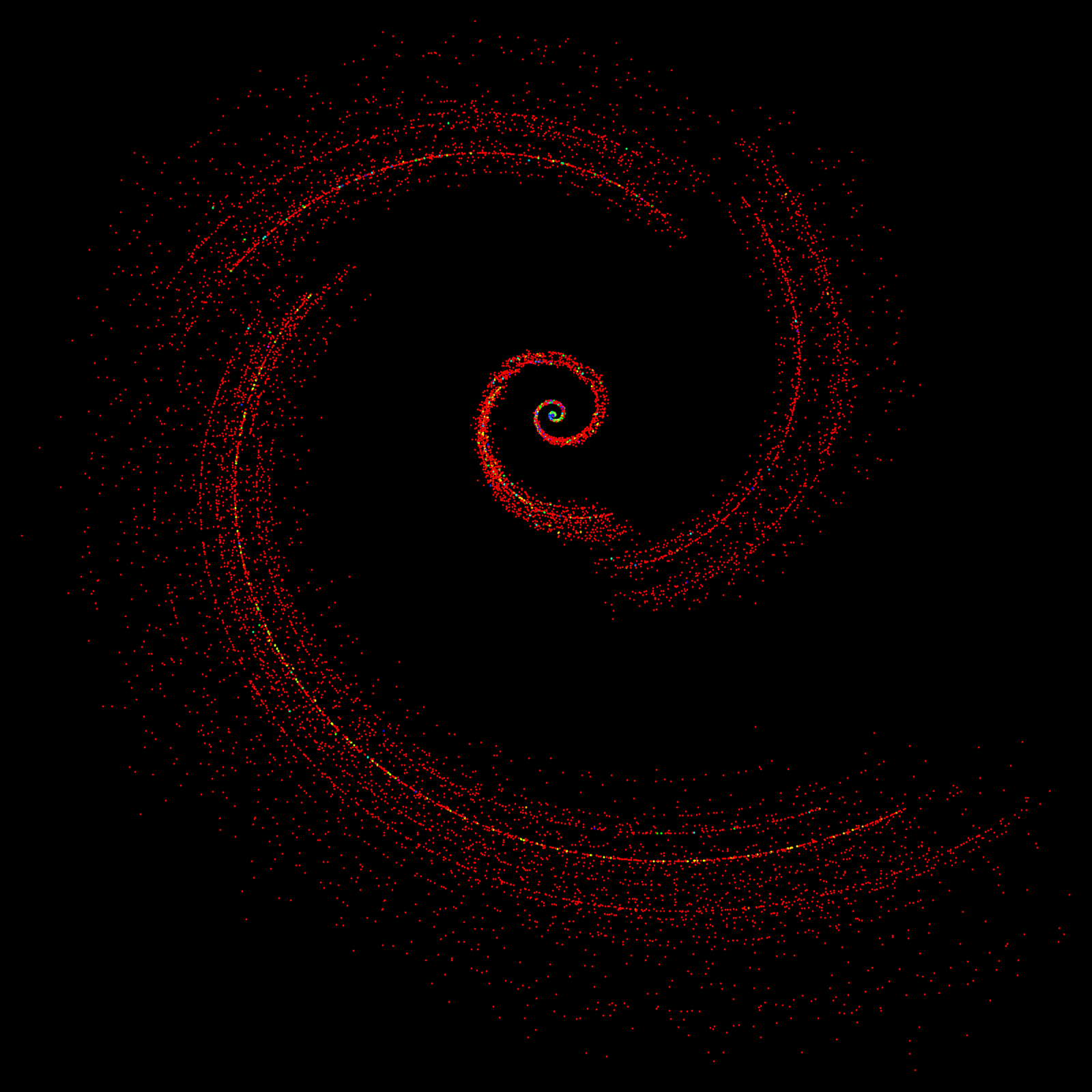M A T H 2 1 B
Mathematics Math21b Spring 2015
Linear Algebra and Differential Equations
Exhibit: GCD spectrum
Office: SciCtr 432# GCD spectra

The English Mathematician Henry Smith first studied in the article Smith, Henry J. Stephen On the Value of a Certain Arithmetical Determinant. Proc. London Math. Soc. S1-7 no. 1, 208, the GCD matrices. There are closed formulas for the determinants even if the entries A(i,j) are defined as gcd(i,j)s, where s is a complex number. In the project, the class had to experiment a bit with computing the spectra. An example of such a matrix (with s=1) is
A= | 1 1 1 1 |
| 1 2 1 2 |
| 1 1 3 1 |
| 1 2 1 4 |
where

det(A) = phi(1) phi(2) phi(3) phi(4) = 4.

Some properties of these determinants were rediscovered by Juan Jose Alba Gonzalez and were communicated to Omar Antolin, who showed it to Oliver. The GCD matrices have so become a bit of a fixture (or rather serious running joke) in the course. We have seen them in the homework, the Mathematica project as well as the final exam (PDF). Here is the code in the project for displaying the spectrum:
GCDMatrix[n_, s_] := Table[GCD[i, j]^s, {i, n}, {j, n}];
e=Eigenvalues[GCDMatrix[1000,1.0+4 I]];
S=Graphics[{PointSize[0.001], Map[complextopoint,e]}]

While working on the project, two students (Isabelle Steinhaus and Jerry Nelluvelili) tried out the parameter s = 1+4i and saw the picture shown at the end. It looks as if these spectra could be interesting. Similarly as Julia sets in complex dynamics, one can look at the corresponding spectrum for each s. Nothing seems to be known. One would like to know for example whether in the limit n to infinity, the spectrum converges to some fractal set. As we have seen in the Mathematica project, there are formulas for the determinant of An(s) in terms of Jordan totient functions which are of very number theoretic nature as det(An(s))/ns is a product of factors (1-1/ps), where p runs over all primes dividing n. The famous Riemann zeta function zeta(s) has the property that 1/zeta(s) is a product of factors (1-1/p^s) where p runs over all primes. So, in some sense, the determinants of the GCD matrices lurch up to the zeta function if n is the product of the first k primes. In other words, the Riemann zeta function is a limit of determinants. This indicates how exciting determinants are:

 Observation: Let pj is the j'th prime. Then det(An(s)/ns converges for n=prodj pj to infinity to the reciprocal of the Riemann zeta function. At least for Re(s)>1.

The following is far fetches since the Riemann zeta function is only defined for Re(s) smaller or equal to 1 through analytic continuation. Look at
log det(An(s) - s log(n)

Since log(det(A)) is the sum of the logarithms of the eigenvalues which mathematicians call the logarithmic potential of the spectrum at z=0. There is a chance that the spectrum converges in the limit to a measure where the potential is finite.

There is now an interesting question: can one say something about the limit of a suitably normalized logarithmic potential of the spectrum of the GCD matrices? Naively looking at the problem and eying the Riemann hypothesis, we would expect that something interesting could happen on the line Re(s)=1/2.

Note that the roots of the function h(s) = det(An>(s)>-1) are on the line Re(s)=0 because it is a product of terms (1-1/p^s) = (1-exp(-s log(p))) are zero for Re(s)=0 and Im(s) = 2pi/log(p). The fact that the the partial products have little to do with the Riemann zeta function for Re(s) below 1 is an indication why the Riemann hypothesis is hard. One can not see so easily to the left of the line Re(s)=1.

What we have explored experimentally in the Mathematica project is not so much the known determinant but the unknown structure of the eigenvalues of these matrices.

GCDMatrix[n_, s_] := Table[GCD[i, j]^s, {i, n}, {j, n}];
JordanFunction[n_, s_] :=
Module[{p = Divisors[n]}, P = {};
Do[If[PrimeQ[p[[i]]], P = Append[P, p[[i]]]], {i, Length[p]}];
n^s Product[1 - 1/P[[j]]^s, {j, 1, Length[P]}]];
JordanTotient[n_, s_] := Product[JordanFunction[j, s], {j, n}];
{JordanTotient[10, 1.0*I], Det[GCDMatrix[10, 1.0*I]]}

We run it for a 10'000 x 10'000 GCD matrix: